Question

Give the electron group geometry and molecular geometry for PC13 tetrahedral, bent trigonal planar, bent tetrahedral,...Give the electron group geometry and molecular geometry for PC13 tetrahedral, bent trigonal planar, bent tetrahedral, trigonal pyramidal trigonal planar, pyramidal trigonal pyramidal, see saw tetrahedral, T-shapted

We need at least 10 more requests to produce the answer.

0 / 10 have requested this problem solution

The more requests, the faster the answer.

All students who have requested the answer will be notified once they are available.

Earn Coins

Coins can be redeemed for fabulous gifts.

Similar Homework Help Questions
• What is the molecular geometry of SO32-? View Available Hint(s) trigonal planar trigonal pyramidal tetrahedral bent...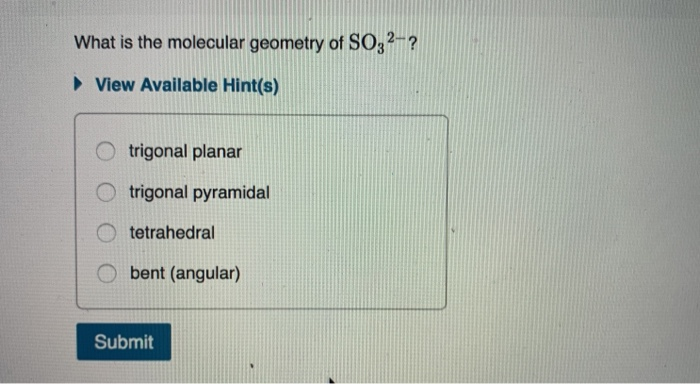What is the molecular geometry of SO32-? View Available Hint(s) trigonal planar trigonal pyramidal tetrahedral bent (angular)

• . Determine the electron geometry (eg), molecular geometry (mg), and polarity of PCl3. eg=tetrahedral, mg=trigonal pyramidal,...

. Determine the electron geometry (eg), molecular geometry (mg), and polarity of PCl3. eg=tetrahedral, mg=trigonal pyramidal, polar eg=tetrahedral, mg=bent, polar eg=linear, mg=linear, nonpolar eg=trigonal planar, mg=trigonal planar, nonpolar eg=trigonal pyramidal, mg=trigonal pyramidal, polar

• The molecular geometry of water is, respectively: Show Work on Scratch Paper! Trigonal planar Tetrahedral Trigonal...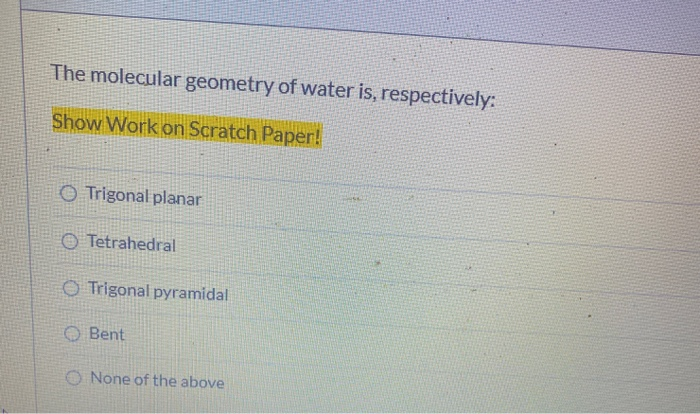The molecular geometry of water is, respectively: Show Work on Scratch Paper! Trigonal planar Tetrahedral Trigonal pyramidal Bent O None of the above

• Determine the molecular geometries of the following ions: SCN^-Molecular geometry: linear tetrahedral bent trigonal pyramidal trigonal...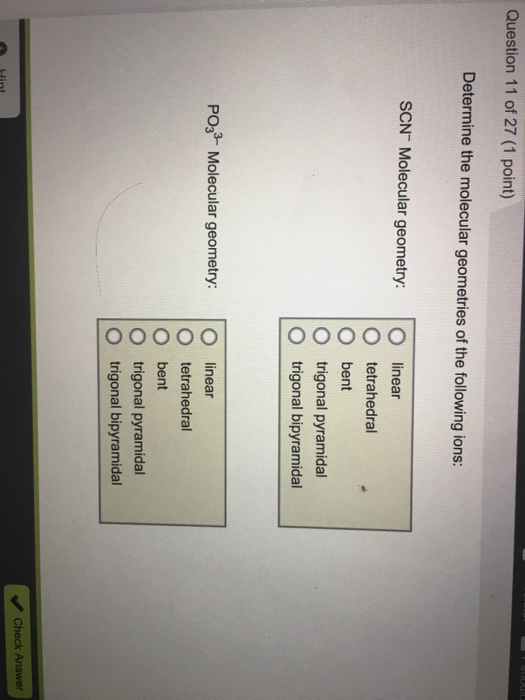Determine the molecular geometries of the following ions: SCN^-Molecular geometry: linear tetrahedral bent trigonal pyramidal trigonal bipyramidal PO_3^3- Molecular geometry: linear tetrahedral bent trigonal pyramidal trigonal bipyramidal

• Determine the electron geometry (eg) and molecular geometry (mg) of PF5. eg=trigonal planar, mg=octahedral eg=tetrahedral, mg=trigonal...

Determine the electron geometry (eg) and molecular geometry (mg) of PF5. eg=trigonal planar, mg=octahedral eg=tetrahedral, mg=trigonal pyramidal eg=trigonal bipyramidal, mg=tetrahedral eg=trigonal bipyramidal, mg=trigonal bipyramidal eg=octahedral, mg=octahedral

• What is the molecular geometry of AsCl3? T-shaped tetrahedral trigonal planar trigonal pyramidal

What is the molecular geometry of AsCl3? T-shaped tetrahedral trigonal planar trigonal pyramidal

• Indicate the electron pair geometry and the molecular geometry for each of the six compounds listed...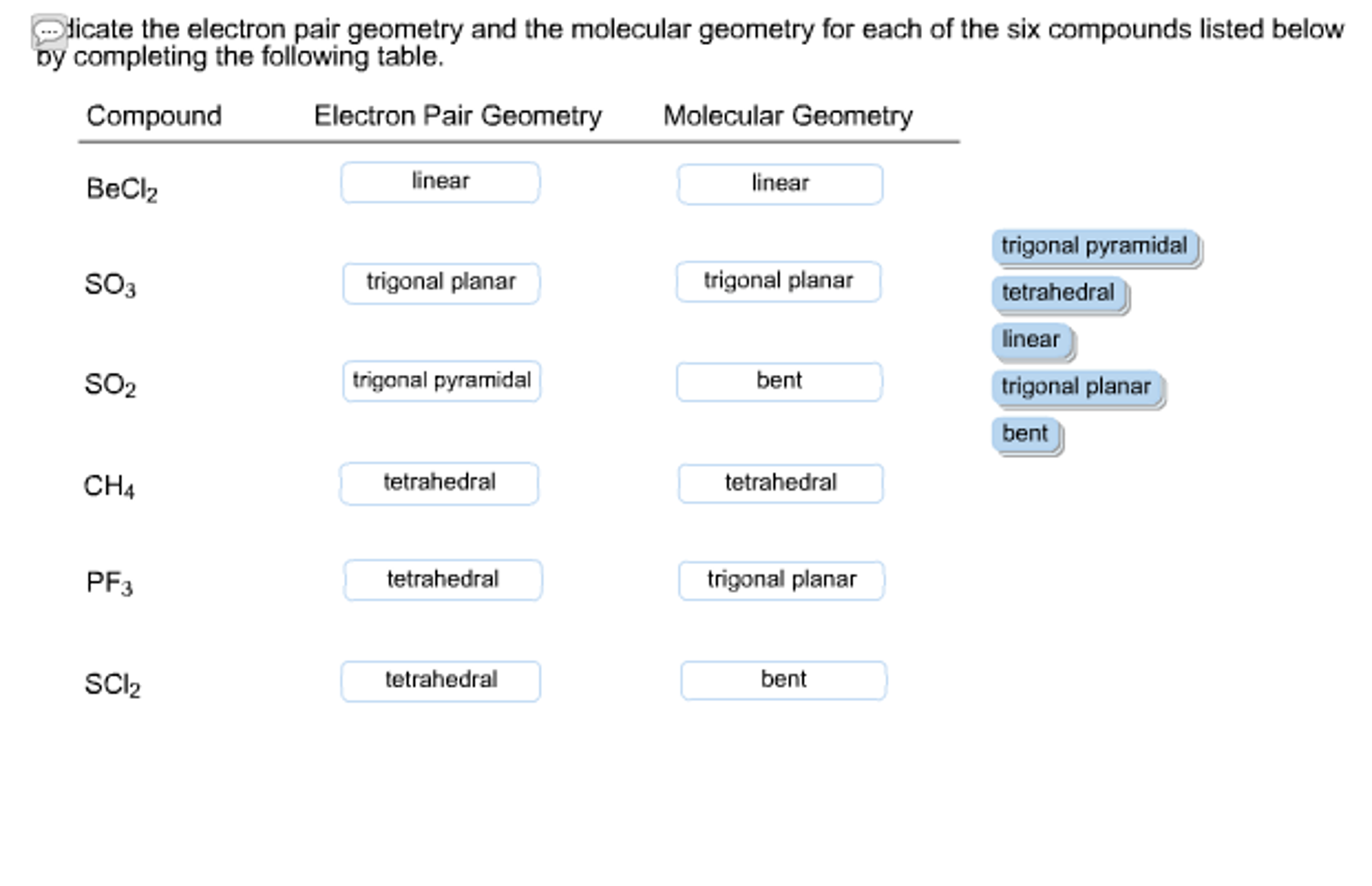Indicate the electron pair geometry and the molecular geometry for each of the six compounds listed below by completing the following table. what did i do wrong?! dicate the electron pair geometry and the molecular geometry for each of the six compounds listed below oy completing the folowing table. Compound Electron Pair Geometry Molecular Geometry BeClz inear linear trigonal pyramidal tetrahedral inear trigonal planar bent SO3 trigonal planar trigonal planar SO2 rigonal pyramidal bent CH4 tetrahedral tetrahedral PF3 tetrahedral trigonal...

• 18. Determine the electron geometry of CBrs C) trigonal planar D) trigonal pyramidal A) linearB) tetrahedral...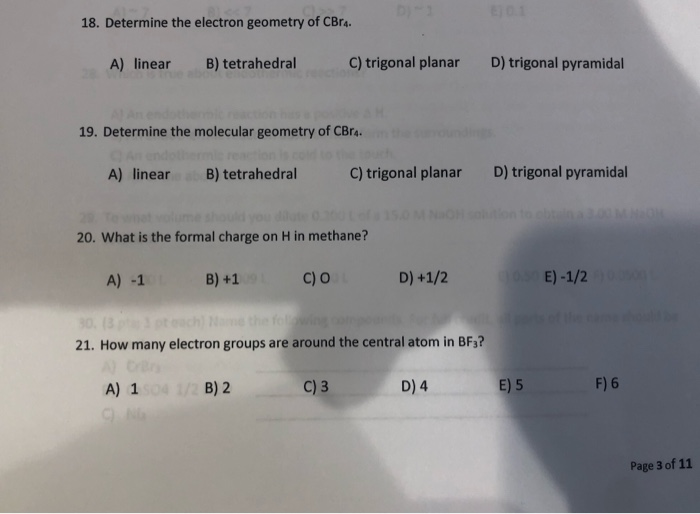18. Determine the electron geometry of CBrs C) trigonal planar D) trigonal pyramidal A) linearB) tetrahedral 19. Determine the molecular geometry of CBra D) trigonal pyramidal C) trigonal planar A) linearB) tetrahedral 20. What is the formal charge on H in methane? 21. How many electron groups are around the central atom in BF? F) 6 D) 4 C)3 A) 1 B) 2 Page 3 of 11

• Determine the electron geometry (eg), molecular geometry(mg) and polarity of XeO3. eg=octahedral, mg=tetrahedral, nonpolar eg=tetrahedral, mg=trigonal...

Determine the electron geometry (eg), molecular geometry(mg) and polarity of XeO3. eg=octahedral, mg=tetrahedral, nonpolar eg=tetrahedral, mg=trigonal pyramidal, polar eg=trigonal planar, mg=trigonal planar, nonpolar eg=trigonal planar, mg=trigonal pyramidal, polar eg=trigonal bipyramidal, mg=trigonal planar, nonpolar

• RESULTS (The choices of electron pair geometry and molecular shape are linear, bent, trigonal planar, trigonal...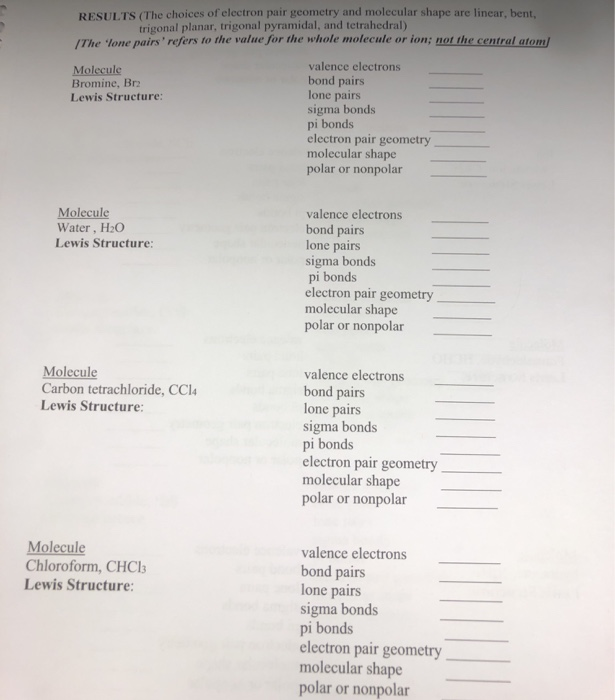RESULTS (The choices of electron pair geometry and molecular shape are linear, bent, trigonal planar, trigonal pyramidal, and tetrahedral) /The lone pairs' refers to the value for the whole molecule or ion; not the central atom/ valence electrons Molecule Bromine, Br bond pairs lone pairs sigma bonds pi bonds electron pair geometry molecular shape polar or nonpolar Lewis Structure: Molecule Water, H2O Lewis Structure: valence electrons bond pairs lone pairs sigma bonds pi bonds electron pair geometry molecular shape polar...